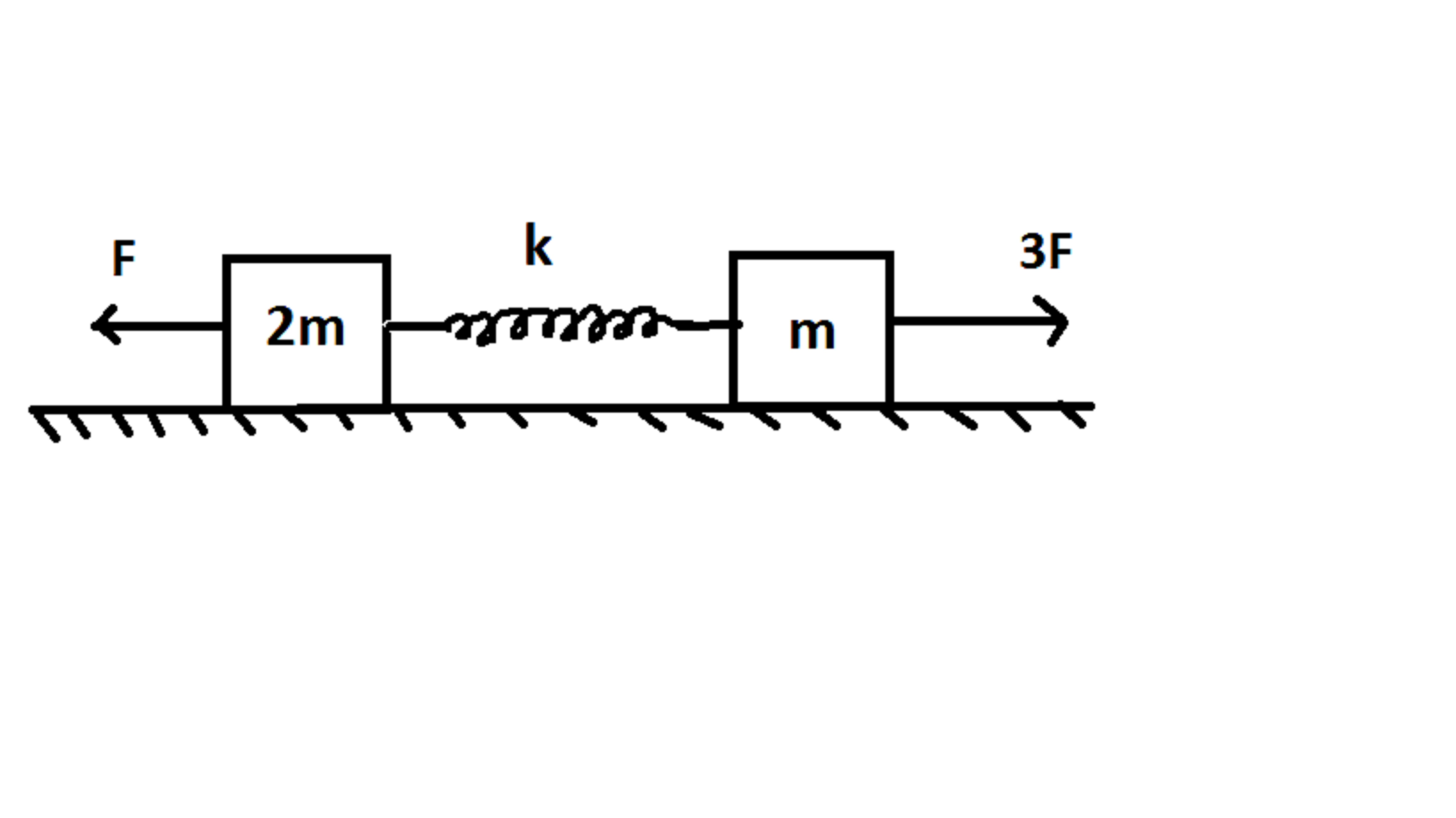In the figure shown above. the surfaces are friction-less. Find the $\text{Maximum}$ extension in the spring(in$\text{ meters}$) , if the blocks are initially at rest and the spring is initially in its natural length.

Details and Assumptions:

$\bullet$ $m= 5kg$

$\bullet$ $F= 30N$

$\bullet$ $k= 700Nm^{-1}$

×